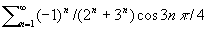# Faton M. Berisha and Muharrem Q. Berisha

### Faculty of Mathematical and Natural Sciences, University of Prishtina, Prishtina,Kosova

For a sequence of real or complex numbersand a given sequencethe linear operatoris defined byTheorem 1. Letbe a convergent real or complex trigonometric series anda sequence of real or complex numbers such that. Then the following equation holds true(1)

where are.

Corollary 1. If there exist the finite limits, then series on the left-hand side of (1) converges faster then the series

on the right-hand side.

The acceleration of convergence is increased with the value of p.

Example 1. Let. ThenObviously, for every integer p the sequencesatisfies the conditions of Corollary 1. In particular, in order to calculate the approximate sum of the serieswith an error not greater thanwe must compute the sum of first 9 terms. Applying the transformation (1), the same accuracy is obtained by computing the sum of 5 terms for, 3 terms forand 1 term for.